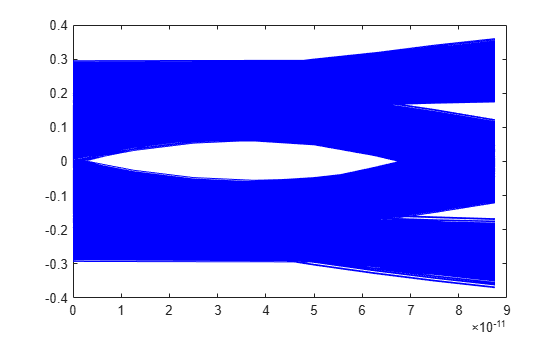# serdes.DFECDR

Decision feedback equalizer (DFE) with clock and data recovery (CDR)

Since R2019a

## Description

The `serdes.DFECDR` System object™ adaptively processes a sample-by-sample input signal or analytically processes an impulse response vector input signal to remove distortions at post-cursor taps.

The DFE modifies baseband signals to minimize the intersymbol interference (ISI) at the clock sampling times. The DFE samples data at each clock sample time and adjusts the amplitude of the waveform by a correction voltage.

For impulse response processing, the hula-hoop algorithm is used to find the clock sampling locations. The zero-forcing algorithm is then used to determine the N correction factors necessary to have no ISI at the N subsequent sampling locations, where N is the number of DFE taps.

For sample-by-sample processing, the clock recovery is accomplished by a first order phase tracking model. The bang-bang phase detector utilizes the unequalized edge samples and equalized data samples to determine the optimum sampling location. The DFE correction voltage for the N-th tap is adaptively found by finding a voltage that compensates for any correlation between two data samples spaced by N symbol times. This requires a data pattern that is uncorrelated with the channel ISI for correct adaptive behavior.

To equalize the input signal:

1. Create the `serdes.DFECDR` object and set its properties.

2. Call the object with arguments, as if it were a function.

## Creation

### Syntax

``dfecdr = serdes.DFECDR``
``dfecdr = serdes.DFECDR(Name,Value)``

### Description

````dfecdr = serdes.DFECDR` returns a DFECDR object that modifies an input waveform with the DFE and determines the clock sampling times. The system object estimates the data symbol according to the Bang-Bang CDR algorithm.```
````dfecdr = serdes.DFECDR(Name,Value)` sets properties using one or more name-value pairs. Enclose each property name in quotes. Unspecified properties have default values.Example: `dfecdr = serdes.DFECDR('Mode',1)` returns a DFECDR object that applies specified DFE tap weights to input waveform.```

## Properties

expand all

Unless otherwise indicated, properties are nontunable, which means you cannot change their values after calling the object. Objects lock when you call them, and the `release` function unlocks them.

If a property is tunable, you can change its value at any time.

### DFE Properties

DFE operating mode, specified as `0`, `1`, or `2`. The operating mode you select determines the DFE tap weights values that the object applies to the input waveform.

Mode ValueDFE ModeDFE Operation
`0`OffThe object bypasses DFE and the input waveform remains unchanged.
`1`FixedThe object applies the DFE tap weights specified in `TapWeights` to the input waveform.
`2`AdaptThe object determines the optimum DFE tap weights and applies them to the input waveform.

Data Types: `double`

Initial DFE tap weights, specified as a row vector in volts. The length of the vector determines the number of taps. The vector elements signify the tap weights or tap strength. Set the tap weight to zero to initialize the tap.

Data Types: `double`

Minimum value of the adapted tap weights, specified as a real scalar or a real-valued row vector in volts. Specify as a scalar to apply to apply the same minimum value to all the DFE taps, or specify as a vector that has the same length as the `TapWeights`.

Data Types: `double`

Maximum value of the adapted tap weights, specified as a nonnegative real scalar or a nonnegative real-valued row vector in volts. Specify as a scalar to apply the same maximum value to all the DFE taps, or specify as a vector that has the same length as the `TapWeights`.

Data Types: `double`

Controls for update rate of tap weights, specified as a unitless nonnegative real scalar. Increase the value of this property for the DFE adaptation to converge faster at the expense of more noise in the DFE tap values.

Data Types: `double`

DFE adaptive step resolution, specified as a nonnegative real scalar or a nonnegative real-valued row vector in volts. Specify as a scalar to apply the same value to all the DFE taps, or specify as a vector that has the same length as `TapWeights`.

`EqualizationStep` specifies the minimum change in DFE taps from one time step to the next to mimic hardware limitations. Setting `EqualizationStep` to zero yields DFE tap values without any resolution limitation.

Data Types: `double`

Multiply DFE tap weights by a factor of two, specified as true or false. Set this property to true to multiply the DFE tap weights by a factor of two.

The output of the slicer in the `serdes.DFECDR` System object from the SerDes Toolbox™ is [-0.5 0.5]. But some industry applications require the slicer output to be [-1 1]. `Taps2x` allows you to quickly double the DFE tap weights to change the slicer reference.

### CDR Properties

Early or late CDR count threshold to trigger a phase update, specified as a unitless real positive integer greater than 4. Increasing the value of `Count` provides a more stable output clock phase at the expense of convergence speed. Because the bit decisions are made at the clock phase output, a more stable clock phase has a better bit error rate (BER).

`Count` also controls the bandwidth of the CDR which is approximately calculated by using the equation:

Data Types: `double`

Clock phase resolution, specified as a real scalar in fraction of symbol time. `ClockStep` is the inverse of the number of phase adjustments in CDR.

Data Types: `double`

Clock phase offset, specified as a real scalar in the range [−0.5, 0.5] in fraction of symbol time. `PhaseOffset` is used to manually shift the clock probability distribution function (PDF) for better BER.

Data Types: `double`

Reference clock offset impairment, specified as a real scalar in the range [−300, 300] in parts per million (ppm). `ReferenceOffset` is the deviation between transmitter oscillator frequency and receiver oscillator frequency.

Data Types: `double`

Sampling latch metastability voltage, specified as a real scalar in volts (V). If the data sample voltage lies within the region (±`Sensitivity`), there is a 50% probability of bit error.

Data Types: `double`

Clock phase detector option used in the clock data recovery. You can choose between bang-bang (Alexander) or baud-rate type-A (Mueller-Muller).

Time of a single symbol duration, specified as a real scalar in seconds (s).

Data Types: `double`

Uniform time step of the waveform, specified as a real scalar in seconds (s).

Data Types: `double`

Modulation scheme, specified as `2`, `3` or `4`.

Modulation ValueModulation Scheme
`2`Non-return to zero (NRZ)
`3`Three-level pulse amplitude modulation (PAM3)
`4`Four-level pulse amplitude modulation (PAM4)

Note

IBIS does not support a PAM3 modulation scheme, so you cannot export the System object to IBIS-AMI model if you set the modulation scheme to PAM3.

Data Types: `double`

Input waveform type, specified as one of these:

• `'Sample'` — A sample-by-sample input signal.

• `'Impulse'` — An impulse-response input signal.

Data Types: `char`

## Usage

### Syntax

``y = dfecdr(x)``

### Description

``y = dfecdr(x)``

### Input Arguments

expand all

Input baseband signal, specified as a scalar or vector. If you set the `WaveType` property to `'Sample'`, then the input signal is a sample-by-sample signal specified as a scalar. If you set the `WaveType` property to `'Impulse'`, the input signal is an impulse-response vector signal.

### Output Arguments

expand all

Estimated channel output. If the input signal is a sample-by-sample signal specified as a scalar, then the output is also scalar. If the input signal is an impulse response vector signal, the output is also a vector.

Estimated DFE tap weight values, returned as a vector.

Relative recovered clock phase, returned as a units of SymbolTime in the range [0,1].

AMI clock bus, returned as a structure consisting of:

• ClockTime — Time taken to sample the data signal.

• clockValidOnRising — Valid clock time value on the rising edge of a signal

Bus containing additional interior CDR signals, returned as a structure consisting of:

• clockPhase — Relative clock phase in units of SymbolTime in the range of [0,1].

• symbolRecovered — Symbol recovered from data signal at ClockTime.

• voltageSample — Voltage observed from the data signal at ClockTime.

• PAM4Threshold — The estimated upper eye at PAM4 threshold.

• CDRedgeVoltage — The voltage observed from the data signal at ClockTime — SymbolTime/2.

• CDRCounter — The bang-bang CDR internal counter used to trigger samples.

• CDREarlyLateCounter — The bang-bang CDR accumulated (or filtered) signal used to trigger updated to the CDR phase.

• PAM4SymbolMiddleVoltage — Estimated PAM4 symbol voltage of the inner eye outer envelope to estimate PAM4 threshold.

• PAM4OuterVoltage — Estimated PAM4 outer envelope voltage to estimate PAM4 threshold.

• EyeHeightAbsAve — Estimates eye height.

## Object Functions

To use an object function, specify the System object as the first input argument. For example, to release system resources of a System object named `obj`, use this syntax:

`release(obj)`

expand all

 `step` Run System object algorithm `release` Release resources and allow changes to System object property values and input characteristics `reset` Reset internal states of System object

## Examples

collapse all

This example shows how to process impulse response of a channel using `serdes.DFECDR` system object™.

Use a symbol time of `100` ps. There are `16` samples per symbol. The channel has `14` dB loss.

```SymbolTime = 100e-12; SamplesPerSymbol = 16; dbloss = 14; NumberOfDFETaps = 2;```

Calculate the sample interval.

`dt = SymbolTime/SamplesPerSymbol; `

Create the DFECDR object. The object adaptively applies optimum DFE tap weights to input impulse response.

```DFE1 = serdes.DFECDR('SymbolTime',SymbolTime,'SampleInterval',dt,... 'Mode',2,'WaveType','Impulse','TapWeights',zeros(NumberOfDFETaps,1));```

Create the channel impulse response.

```channel = serdes.ChannelLoss('Loss',dbloss,'dt',dt,... 'TargetFrequency',1/SymbolTime/2); impulseIn = channel.impulse;```

Process the impulse response with DFE.

`[impulseOut,TapWeights] = DFE1(impulseIn);`

Convert the impulse response to a pulse, a waveform and an eye diagram for visualization.

```ord = 6; dataPattern = prbs(ord,2^ord-1)-0.5; pulseIn = impulse2pulse(impulseIn,SamplesPerSymbol,dt); waveIn = pulse2wave(pulseIn,dataPattern,SamplesPerSymbol); eyeIn = reshape(waveIn,SamplesPerSymbol,[]); pulseOut = impulse2pulse(impulseOut,SamplesPerSymbol,dt); waveOut = pulse2wave(pulseOut,dataPattern,SamplesPerSymbol); eyeOut = reshape(waveOut,SamplesPerSymbol,[]);```

Create the time vectors.

```t = dt*(0:length(pulseOut)-1)/SymbolTime; teye = t(1:SamplesPerSymbol); t2 = dt*(0:length(waveOut)-1)/SymbolTime;```

Plot the resulting waveforms.

```figure plot(t,pulseIn,t,pulseOut) legend('Input','Output') title('Pulse Response Comparison') xlabel('SymbolTimes'),ylabel('Voltage') grid on axis([41 55 -0.1 0.4]) ```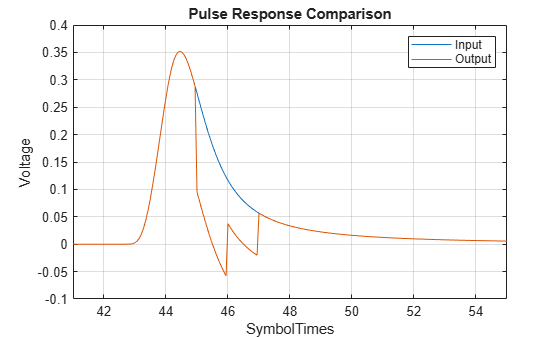```figure plot(t2,waveIn,t2,waveOut) legend('Input','Output') title('Waveform Comparison') xlabel('SymbolTimes'),ylabel('Voltage') grid on ```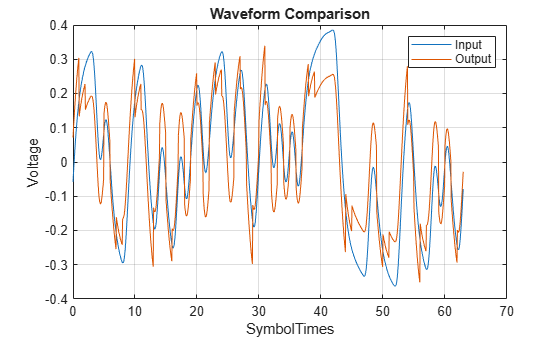```figure subplot(211),plot(teye,eyeIn,'b') xlabel('SymbolTimes'),ylabel('Voltage') grid on title('Input Eye Diagram') subplot(212),plot(teye,eyeOut,'b') xlabel('SymbolTimes'),ylabel('Voltage') grid on title('Output Eye Diagram')```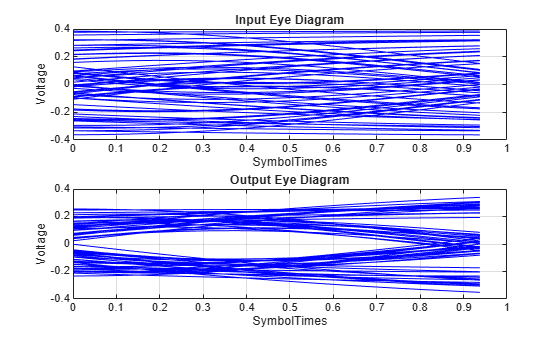This example shows how to process impulse response of a channel one sample at a time using `serdes.DFECDR` System object™.

Use a symbol time of `100` ps, with `8` samples per symbol. The channel loss is `14` dB. Select `12`-th order pseudorandom binary sequence (PRBS), and simulate the first `20000` symbols.

```SymbolTime = 100e-12; SamplesPerSymbol = 8; dbloss = 14; NumberOfDFETaps = 2; prbsOrder = 12; M = 20000; ```

Calculate the sample interval.

`dt = SymbolTime/SamplesPerSymbol;`

Create the DFECDR System object. Process the channel one sample at a time by setting the input waveforms to `'sample'` type. The object adaptively applies the optimum DFE tap weights to input waveform.

```DFE2 = serdes.DFECDR('SymbolTime',SymbolTime,'SampleInterval',dt,... 'Mode',2,'WaveType','Sample','TapWeights',zeros(NumberOfDFETaps,1),... 'EqualizationStep',0,'EqualizationGain',1e-3);```

Create the channel impulse response.

```channel = serdes.ChannelLoss('Loss',dbloss,'dt',dt,... 'TargetFrequency',1/SymbolTime/2);```

Initialize the PRBS generator.

`[dataBit,prbsSeed]=prbs(prbsOrder,1);`

Generate the sample-by-sample eye diagram.

```%Loop through one symbol at a time. inSymbol = zeros(SamplesPerSymbol,1); outWave = zeros(SamplesPerSymbol*M,1); dfeTapWeightHistory = nan(M,NumberOfDFETaps); for ii = 1:M %Get new symbol [dataBit,prbsSeed]=prbs(prbsOrder,1,prbsSeed); inSymbol(1:SamplesPerSymbol) = dataBit-0.5; %Convolve input waveform with channel y = channel(inSymbol); %Process one sample at a time through the DFE for jj = 1:SamplesPerSymbol [outWave((ii-1)*SamplesPerSymbol+jj),TapWeights] = DFE2(y(jj)); end %Save DFE taps dfeTapWeightHistory(ii,:) = TapWeights; end```

```figure plot(dfeTapWeightHistory) grid on legend('TapWeights(1)','TapWeights(2)') xlabel('Symbols') ylabel('Voltage') title('DFE Taps') ```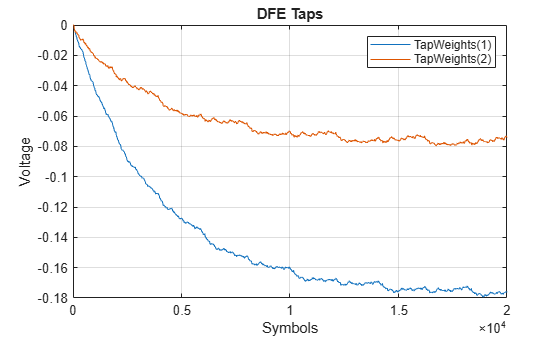You can observe from the plot that the DFE adaptation is approximately complete after the first `10000` symbols, so these can be truncated from the array. Then plot the eye diagram by applying the reshape function to the array of symbols.

```foldedEye = reshape(outWave(10000*SamplesPerSymbol+1:M*SamplesPerSymbol),SamplesPerSymbol,[]); t = dt*(0:SamplesPerSymbol-1); figure,plot(t,foldedEye,'b');```